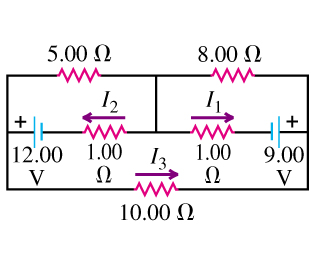# Problem: Calculate the three currents I1,I2, and I3 indicated in the circuit diagram shown in the figure (Figure 1) .

###### FREE Expert Solution

We need Kirchhoff's junction rule to solve this problem.

Kirchhoff's junction rule states that current INTO a junction is always EQUAL to current OUT of the junction.

That is,

$\overline{){\mathbf{\sum }}{{\mathbf{I}}}_{\mathbf{i}\mathbf{n}}{\mathbf{=}}{\mathbf{\sum }}{{\mathbf{I}}}_{\mathbf{o}\mathbf{u}\mathbf{t}}}$

We'll also use Kirchoff's loop rule, which is written as:

$\overline{){\mathbf{\Sigma }}{\mathbf{V}}{\mathbf{=}}{\mathbf{0}}}$

We also need to know, from Ohm's law, that:

$\overline{){\mathbf{V}}{\mathbf{=}}{\mathbf{I}}{\mathbf{R}}}$

We can get the current through the 5.00-Ω and 8.00-Ω resistors in terms of I1, I2, and I3 using the junction rule.

We then apply Kirchhoff's loop rule to the three loops visible in the circuit.

92% (476 ratings)###### Problem Details

Calculate the three currents I1,I2, and I3 indicated in the circuit diagram shown in the figure (Figure 1) .Frequently Asked Questions

What scientific concept do you need to know in order to solve this problem?

Our tutors have indicated that to solve this problem you will need to apply the Kirchhoff's Loop Rule concept. You can view video lessons to learn Kirchhoff's Loop Rule. Or if you need more Kirchhoff's Loop Rule practice, you can also practice Kirchhoff's Loop Rule practice problems.